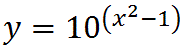Thread: Finding a derivative

1. Finding a derivative

Hello, how do I find dy/dx ofPlease work it out for me to see rather than just give an answer. Thanks!
And also, since I'm still trying to figure out LaTex, will you show me how to display this equation using this coding?

2. Use the derivative for $\displaystyle y=a^x$ and use the chain rule for the index.

3.Originally Posted by Archie MeadeUse the derivative for $\displaystyle y=a^x$ and use the chain rule for the index.
So would it be
2x ln10 10^[(x^2)-1] ?
Please excuse my lack of coding.

4. The $\displaystyle ln10$ will be a denominator, otherwise yes.

5.Originally Posted by Archie MeadeThe $\displaystyle ln10$ will be a denominator, otherwise yes.
his derivative is correct.

$\displaystyle \frac{d}{dx} (a^u) = a^u \cdot \ln{a} \cdot \frac{du}{dx}$

$\displaystyle \int a^u \, du = \frac{a^u}{\ln{a}} + C$

6. yes!
i accidentally gave the version from the integral.

Search Tags

derivative, finding